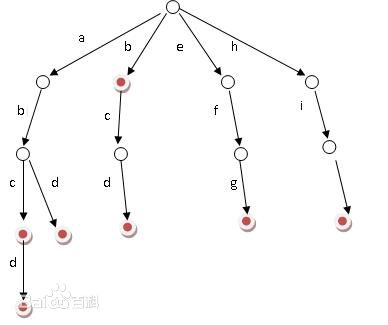# 字典树

(1) 从根结点开始一次搜索；
(2) 取得要查找关键词的第一个字母，并根据该字母选择对应的子树并转到该子树继续进行检索；
(3) 在相应的子树上，取得要查找关键词的第二个字母,并进一步选择对应的子树进行检索。
(4) 迭代过程……
(5) 在某个结点处，关键词的所有字母已被取出，则读取附在该结点上的信息，即完成查找。#define MAX 26
typedef struct TrieNode                     //Trie结点声明

bool isStr;                            //标记该结点处是否构成单词
struct TrieNode *next[MAX];            //儿子分支
}Trie;

①如果当前节点不存在（深度不够），建立节点

②如果构造当前节点的路径（就是单词）未被标记，那么标记改点为单词

#include <iostream>
#include<cstdlib>
#define MAX 26
using namespace std;

typedef struct TrieNode                     //Trie结点声明
{
bool isStr;                            //标记该结点处是否构成单词
struct TrieNode *next[MAX];            //儿子分支
}Trie;

void insert(Trie *root,const char *s)     //将单词s插入到字典树中
{
if(root==NULL||*s=='\0')
return;
int i;
Trie *p=root;
while(*s!='\0')
{
if(p->next[*s-'a']==NULL)        //如果不存在，则建立结点
{
Trie *temp=(Trie *)malloc(sizeof(Trie));
for(i=0;i<MAX;i++)
{
temp->next[i]=NULL;
}
temp->isStr=false;
p->next[*s-'a']=temp;
p=p->next[*s-'a'];
}
else
{
p=p->next[*s-'a'];
}
s++;
}
p->isStr=true;                       //单词结束的地方标记此处可以构成一个单词
}

int search1(Trie *root,const char *s)       //完全对齐
{
Trie *p=root;
while(p!=NULL&&*s!='\0')
{
p=p->next[*s-'a'];
s++;
}
return (p!=NULL&&p->isStr==true);
}

int search2(Trie *root,const char *s)      //头对齐
{
Trie *p=root;
while(p!=NULL&&*s!='\0')
{
if(p->isStr==true)
{
return 1;
}
p=p->next[*s-'a'];
s++;
}

return (p!=NULL&&p->isStr==true);
}

int search3(Trie *root,const char *s)      //尾对齐
{
while(*s!='\0')
{
if(search1(root,s))
return 1;
else
s++;
}
return 0;
}

int search4(Trie *root,const char *s)      //检索
{
while(*s!='\0')
{
if(search2(root,s))
return 1;
else
s++;
}
return 0;
}

void del(Trie *root)                      //释放整个字典树占的堆区空间
{
int i;
for(i=0;i<MAX;i++)
{
if(root->next[i]!=NULL)
{
del(root->next[i]);
}
}
free(root);
}

int main(int argc, char *argv[])
{
int i;
int n,m;                              //n为建立Trie树输入的单词数，m为要查找的单词数
char s;
Trie *root= (Trie *)malloc(sizeof(Trie));
for(i=0;i<MAX;i++)
{
root->next[i]=NULL;
}
root->isStr=false;
scanf("%d",&n);
getchar();
for(i=0;i<n;i++)                 //先建立字典树
{
scanf("%s",s);
insert(root,s);
}
while(scanf("%d",&m)!=EOF)
{
for(i=0;i<m;i++)                 //查找
{
scanf("%s",s);
if(search4(root,s)==1)
{
printf("YES\n");
}
else
printf("NO\n");
}
printf("\n");
}
del(root);                         //释放空间很重要
return 0;
}


int GetCharFromGBK(char gbk_buf)
{
int value=0;
value=gbk_buf;
return value>0?value:value+256;
}

08-245万+
10-21169209-075万+
04-094860
06-284208
11-212496
01-16355
06-22250
07-176536
08-251290
08-041万+
10-16185
08-061397
01-052万+
07-297134